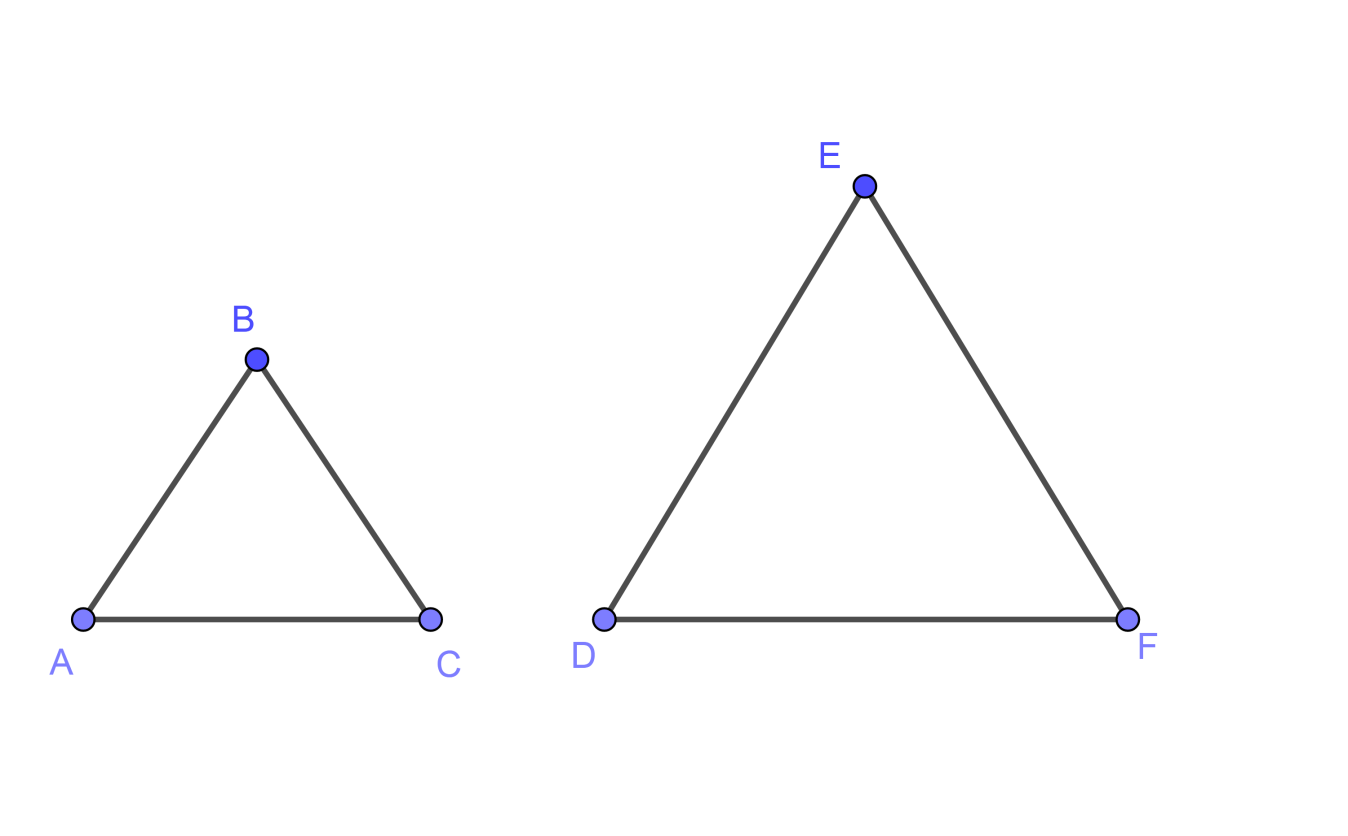Courses
Courses for Kids
Free study material
Free LIVE classes
MoreLIVE
Join Vedantu’s FREE Mastercalss

# If $\Delta ABC\sim \Delta DEF$ such that area of $\Delta ABC$ is $9c{{m}^{2}}$ and the area of $\Delta DEF$ is $16c{{m}^{2}}$ and BC = 2.1 cm. Find the length of EF.Verified
358.5k+ views
Hint: Use the concept of similar triangles i.e. “If two triangles are similar, then the ratio of the area of both triangles is proportional to the square of the ratio of their corresponding sides.” And write the relation between area and corresponding sides of the similar triangles and put the given values in it you will get the answer.

To solve the above problem we should draw the diagram with given notations as follows,Now we will write the given data,
$\Delta ABC\sim \Delta DEF$
Area of $\Delta ABC$ = $A\left( \Delta ABC \right)$ = $9c{{m}^{2}}$
Area of $\Delta DEF$ = $A\left( \Delta DEF \right)$ = $16c{{m}^{2}}$
BC = 2.1 cm.
As the two triangles are similar therefore the relation between their corresponding sides is given by the concept given below,
Concept:
If two triangles are similar then their corresponding sides are in proportion.
By using the above concept we can write,
$\dfrac{AB}{DE}=\dfrac{BC}{EF}=\dfrac{AC}{DE}$ …………………………………………………. (1)
As we have given the value of one of the side BC and there’s no other side given therefore we have to use the area given to find the value of EF and for that we should know the concept given below,
Concept:
If two triangles are similar, then the ratio of the area of both triangles is proportional to the square of the ratio of their corresponding sides.
By using the above concept and squaring the equation (1) we will get,
$\dfrac{A\left( \Delta ABC \right)}{A\left( \Delta DEF \right)}=\dfrac{A{{B}^{2}}}{D{{E}^{2}}}=\dfrac{B{{C}^{2}}}{E{{F}^{2}}}=\dfrac{A{{C}^{2}}}{D{{E}^{2}}}$
Above equation can also be written as,
$\therefore \dfrac{A\left( \Delta ABC \right)}{A\left( \Delta DEF \right)}=\dfrac{B{{C}^{2}}}{E{{F}^{2}}}$
If we put the given values in above equation we will get,
$\therefore \dfrac{9}{16}=\dfrac{{{\left( 2.1 \right)}^{2}}}{E{{F}^{2}}}$
As we know that the square of 2.1 is 4.41 therefore we will get,
$\therefore \dfrac{9}{16}=\dfrac{4.41}{E{{F}^{2}}}$
If we shift the $E{{F}^{2}}$ on the left hand side of the equation we will get,
$\therefore E{{F}^{2}}\times \dfrac{9}{16}=4.41$
If we shift $\dfrac{9}{16}$ on the right hand side of the equation we will get,
$\therefore E{{F}^{2}}=4.41\times \dfrac{16}{9}$
If we do the further simplifications in the above equation we will get,
$\therefore E{{F}^{2}}=7.84$
Now if we take the square roots on both sides of the equations we will get,
$\therefore \sqrt{E{{F}^{2}}}=\sqrt{7.84}$
Therefore, EF = 2.8 cm
Therefore the value of EF is 2.8 cm.

Note: There are chances that you write the relation between area and sides of the similar triangles as, $\dfrac{A\left( \Delta ABC \right)}{A\left( \Delta DEF \right)}=\dfrac{AB}{DE}=\dfrac{BC}{EF}=\dfrac{AC}{DE}$ but you should remember that it is $\dfrac{A\left( \Delta ABC \right)}{A\left( \Delta DEF \right)}=\dfrac{A{{B}^{2}}}{D{{E}^{2}}}=\dfrac{B{{C}^{2}}}{E{{F}^{2}}}=\dfrac{A{{C}^{2}}}{D{{E}^{2}}}$.
Last updated date: 21st Sep 2023
Total views: 358.5k
Views today: 5.58k